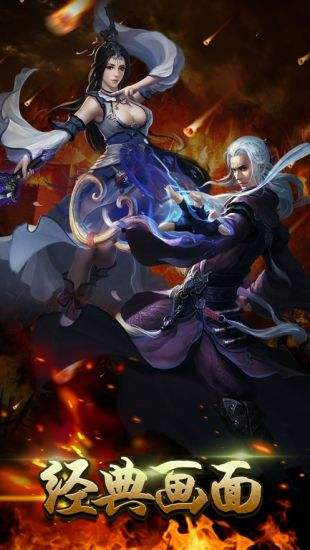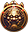元神版传奇世界下载

★散人首推法道，打怪和升级聚灵珠都很快。

★前期首推法战、法道，中后期法战、战战、道道，法师战士可学习独创技能：剑气淬毒。Level41= 500W　　Level47=8000W　 Level53=8.0亿　　 Level59=29.0亿

Level42= 800W　　Level48=1.2亿　　Level54=10.0亿　　Level60=29.0亿

Level43=1200W　　Level49=2.0亿　 Level55=15.0亿

Level44=2400W　　Level50=3.0亿　 Level56=20.0亿

Level45=4000W　　Level51=4.0亿　 Level57=25.0亿

Level46=6000W　　Level52=6.0亿　 Level58=29.0亿

元神版传奇世界下载游戏截图元神版传奇世界下载相关推荐